### C = 60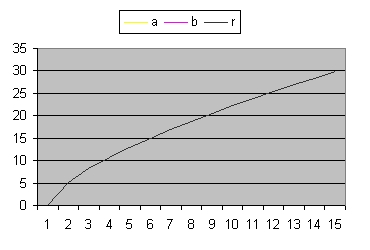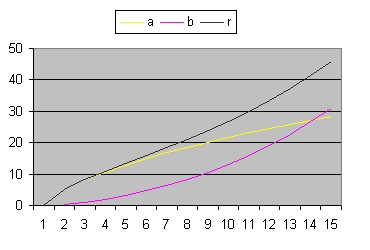Figure 1
Figure 2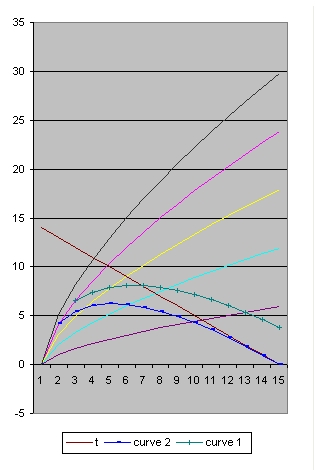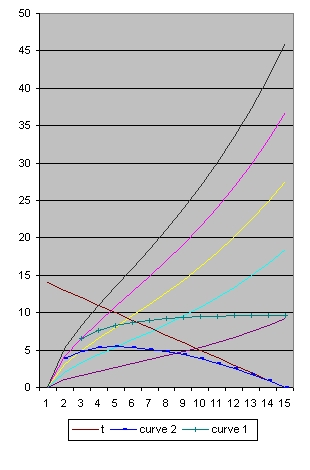Figure 3
Figure 8Figure 4
Figure 9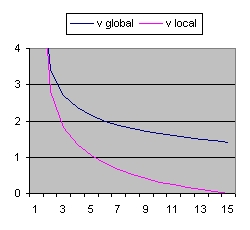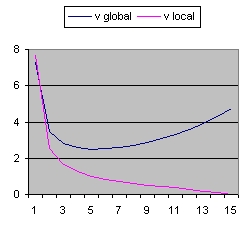Figure 4 A
C=60 Lambda=0
Omega(M) = 0.999952
Rho = 0.001082
Figure 9 A
C=60 Lambda=0.03
Omega(M) = 0.058175
Rho = 0.000295
When you compare the pictures from the left with the right, specific Figure 3 and Figure 8 below the brown line, than they are almost identical.
What that means is that based what is observed (redshift) it is very difficult to distinquish between the two simulations.
Specific what is difficult is to calculate the cosmological constant. The main reason is because the influence locally is very small.

Back to Friedmann's Equation Question 1
Back to Friedmann's Equation Question 3